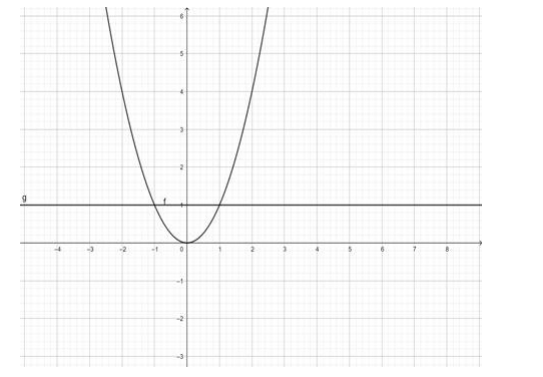# Mark (√) against the correct answer in the following:Question:

Mark (√) against the correct answer in the following:

$f: R \rightarrow R: f(x)=x^{2}$ is

A. one - one and onto

B. one - one and into

C. many - one and onto

D. many - one and into

Solution:

$f(x)=x^{2}$

$\Rightarrow y=x^{2}$

in this range the lines cut the curve in 2 equal valued points of $y$, therefore, the function $f(x)=x^{2}$ is many one.

Range of $f(x)=(0, \infty) \neq R$ (codomain)

$\therefore f(x)$ is into$\therefore f: R \rightarrow R: f(x)=x^{2}$ is many - one into